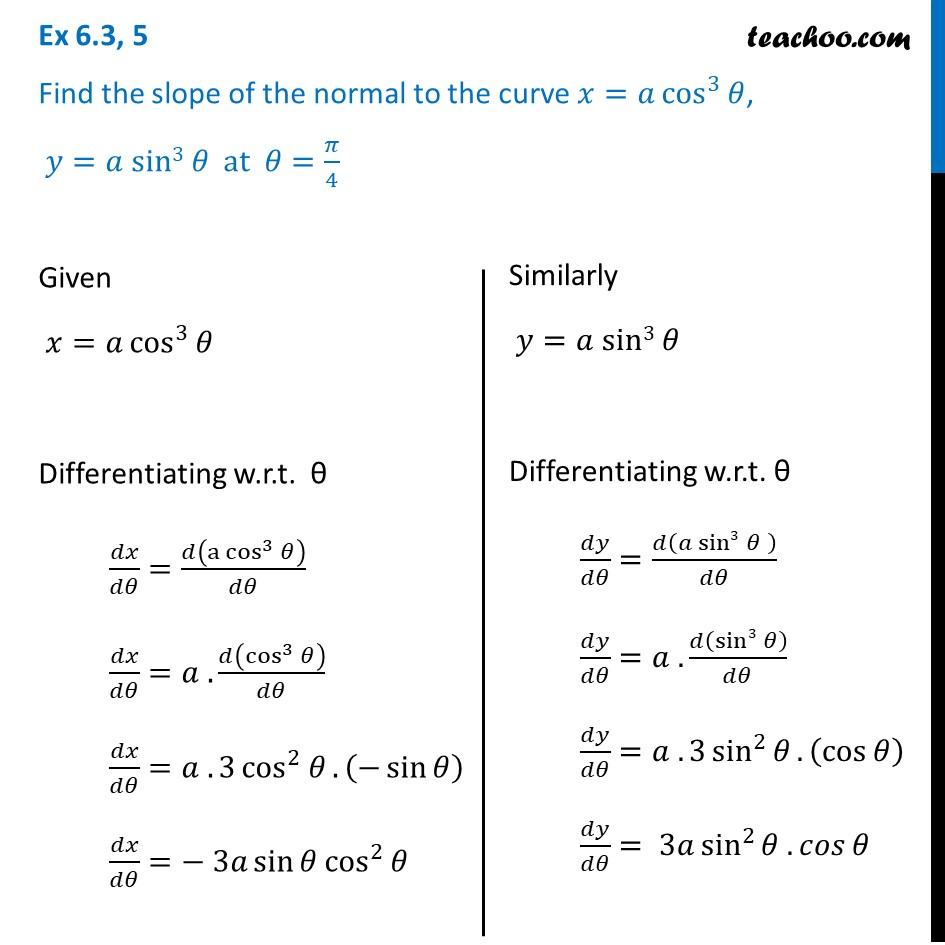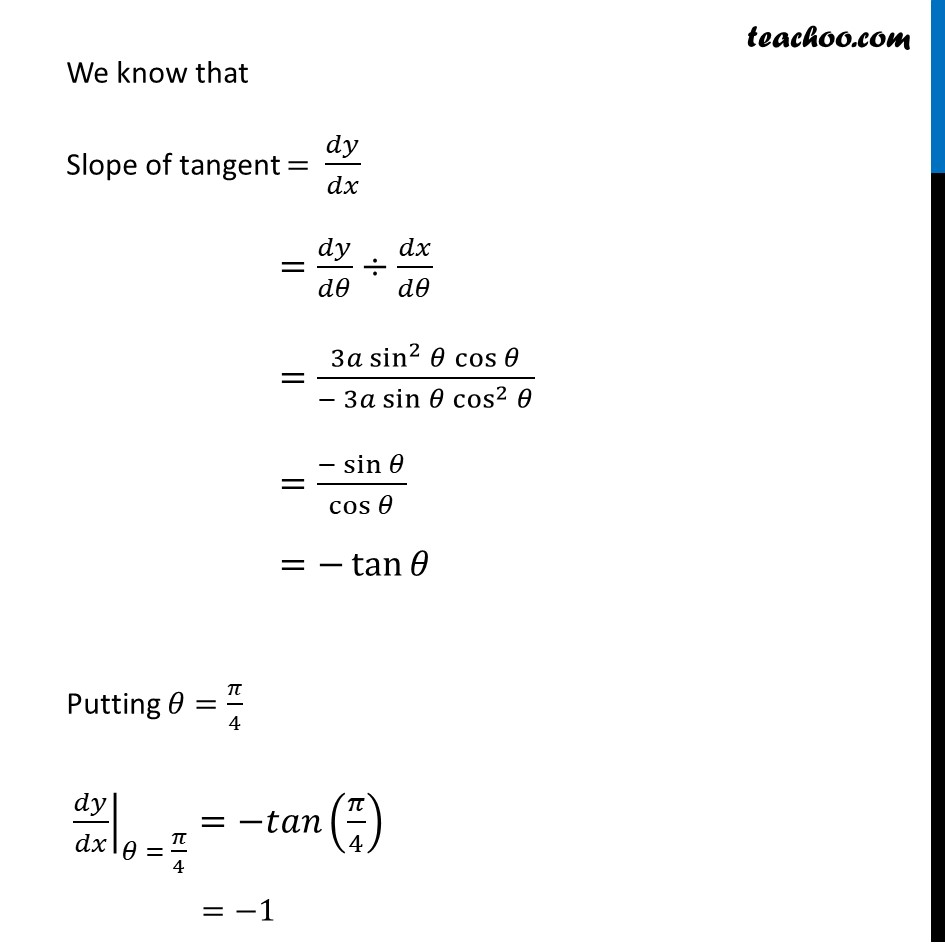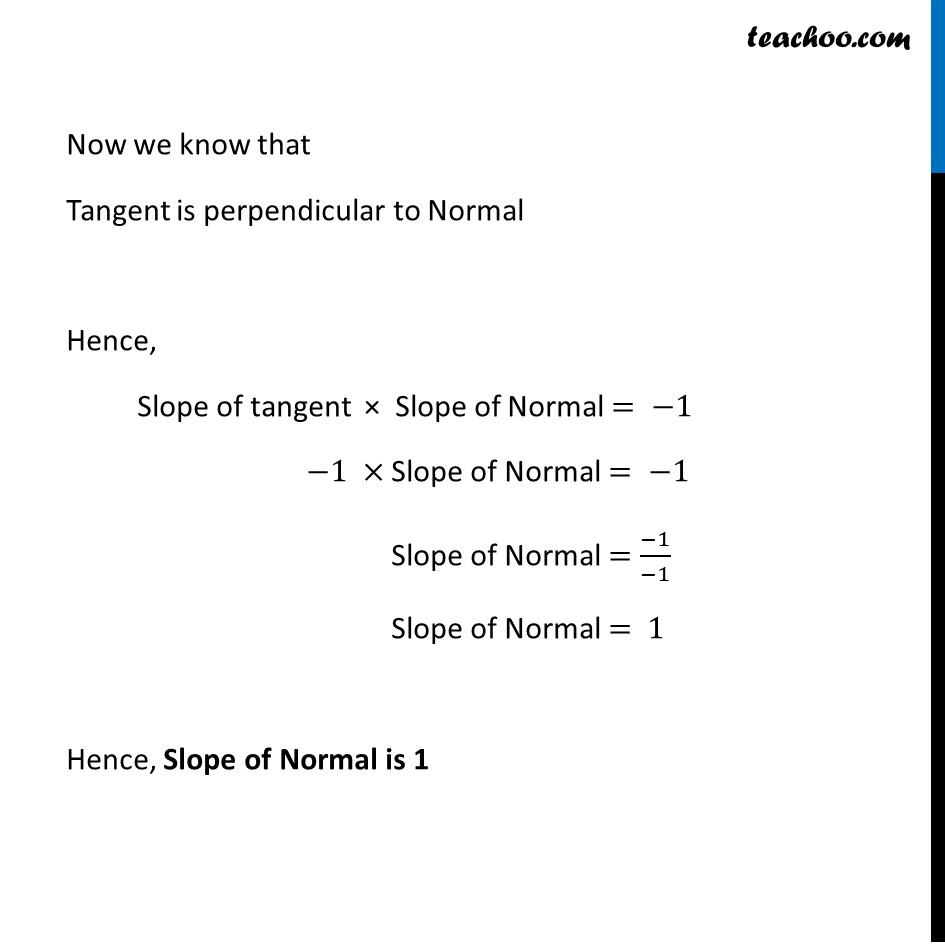Tangents and Normals (using Differentiation)

Chapter 6 Class 12 Application of Derivatives
Serial order wiseLearn in your speed, with individual attention - Teachoo Maths 1-on-1 Class

### Transcript

Question 5 Find the slope of the normal to the curve 𝑥=𝑎 cos^3⁡𝜃, 𝑦=𝑎 sin3 𝜃 at 𝜃=𝜋/4Given 𝑥=𝑎 cos^3⁡𝜃 Differentiating w.r.t. θ 𝑑𝑥/𝑑𝜃=𝑑(〖a cos〗^3⁡𝜃 )/𝑑𝜃 𝑑𝑥/𝑑𝜃=𝑎 .𝑑(cos^3⁡𝜃 )/𝑑𝜃 𝑑𝑥/𝑑𝜃=𝑎 . 3 cos^2⁡𝜃. (−sin⁡𝜃 ) 𝑑𝑥/𝑑𝜃=− 3𝑎 sin⁡〖𝜃 cos^2⁡𝜃 〗 Similarly 𝑦=𝑎 sin3 𝜃 Differentiating w.r.t. θ 𝑑𝑦/𝑑𝜃=𝑑(𝑎 sin3 𝜃" " )/𝑑𝜃 𝑑𝑦/𝑑𝜃=𝑎 .𝑑(sin3 𝜃)/𝑑𝜃 𝑑𝑦/𝑑𝜃=𝑎 . 3 sin^2⁡𝜃. (cos⁡𝜃 ) 𝑑𝑦/𝑑𝜃= 3𝑎 sin^2⁡〖𝜃 .𝑐𝑜𝑠⁡𝜃 〗 We know that Slope of tangent = 𝑑𝑦/𝑑𝑥 =𝑑𝑦/𝑑𝜃÷𝑑𝑥/𝑑𝜃 =(3𝑎 sin^2⁡〖𝜃 cos⁡𝜃 〗)/(− 3𝑎 sin⁡〖𝜃 cos^2⁡𝜃 〗 ) =(−sin⁡𝜃)/cos⁡𝜃 =−tan⁡𝜃 Putting 𝜃=𝜋/4 ├ 𝑑𝑦/𝑑𝑥┤|_(𝜃 = 𝜋/4)=−𝑡𝑎𝑛(𝜋/4) =−1 Now we know that Tangent is perpendicular to Normal Hence, Slope of tangent × Slope of Normal = −1 −1 × Slope of Normal = −1 Slope of Normal =(−1)/(−1) Slope of Normal = 1 Hence, Slope of Normal is 1